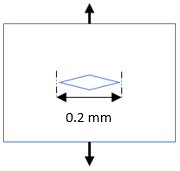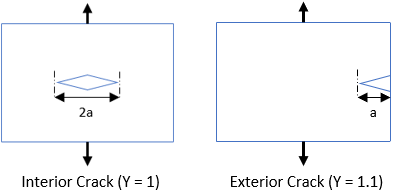## Fracture Stress

A brittle specimen made out of Silicone Carbide has a stress intensity of 3.5 MPa∙m^(1/2). What is the applied tensional force (MPa) if the specimen developed the interior crack shown in the figure?Hint
$$K_{IC}=Y\cdot \sigma \cdot \sqrt{\pi (a)}$$$where $$K_{IC}$$ is fracture toughness, $$\sigma$$ is applied stress, $$a$$ is crack length, and $$Y$$ is geometrical factor. Hint 2Fracture toughness is the stress intensity of when a brittle material will fail due to the combination of an applied stress and crack length. $$K_{IC}=Y\cdot \sigma \cdot \sqrt{\pi (a)}$$$
where $$K_{IC}$$ is fracture toughness, $$\sigma$$ is applied stress, $$a$$ is crack length, and $$Y$$ is geometrical factor.Based on the problem statement:
• $$Y=1$$ (since an interior crack was produced)
• $$K_{IC}=3.5\:MPa\cdot m^{1/2}$$
• $$a=\frac{0.2mm}{2}=0.1\:mm$$
Solving for applied stress:
$$\sigma=\frac{K_{IC}}{Y \sqrt{\pi (a)}}$$$$$\sigma=\frac{3.5\:MPa\cdot\sqrt{ m}}{(1) \sqrt{\pi (0.0001m)}}=\frac{3.5MPa\sqrt{m}}{0.0177\sqrt{m}}=197.5\:MPa$$$
197.5 MPa